2016/01/12 14:35

# The Shapely User Manual

Abstract: This document explains how to use the Shapely Python package for computational geometry.

## Introduction

Deterministic spatial analysis is an important component of computational approaches to problems in agriculture, ecology, epidemiology, sociology, and many other fields. What is the surveyed perimeter/area ratio of these patches of animal habitat? Which properties in this town intersect with the 50-year flood contour from this new flooding model? What are the extents of findspots for ancient ceramic wares with maker’s marks “A” and “B”, and where do the extents overlap? What’s the path from home to office that best skirts identified zones of location based spam? These are just a few of the possible questions addressable using non-statistical spatial analysis, and more specifically, computational geometry.

Shapely is a Python package for set-theoretic analysis and manipulation of planar features using (via Python’s ctypes module) functions from the well known and widely deployed GEOS library. GEOS, a port of the Java Topology Suite (JTS), is the geometry engine of the PostGIS spatial extension for the PostgreSQL RDBMS. The designs of JTS and GEOS are largely guided by the Open Geospatial Consortium‘s Simple Features Access Specification  and Shapely adheres mainly to the same set of standard classes and operations. Shapely is thereby deeply rooted in the conventions of the geographic information systems (GIS) world, but aspires to be equally useful to programmers working on non-conventional problems.

The first premise of Shapely is that Python programmers should be able to perform PostGIS type geometry operations outside of an RDBMS. Not all geographic data originate or reside in a RDBMS or are best processed using SQL. We can load data into a spatial RDBMS to do work, but if there’s no mandate to manage (the “M” in “RDBMS”) the data over time in the database we’re using the wrong tool for the job. The second premise is that the persistence, serialization, and map projection of features are significant, but orthogonal problems. You may not need a hundred GIS format readers and writers or the multitude of State Plane projections, and Shapely doesn’t burden you with them. The third premise is that Python idioms trump GIS (or Java, in this case, since the GEOS library is derived from JTS, a Java project) idioms.

If you enjoy and profit from idiomatic Python, appreciate packages that do one thing well, and agree that a spatially enabled RDBMS is often enough the wrong tool for your computational geometry job, Shapely might be for you.

### Spatial Data Model

The fundamental types of geometric objects implemented by Shapely are points, curves, and surfaces. Each is associated with three sets of (possibly infinite) points in the plane. Theinterior,boundary, andexteriorsets of a feature are mutually exclusive and their union coincides with the entire plane.

• APointhas aninteriorset of exactly one point, aboundaryset of exactly no points, and anexteriorset of all other points. APointhas a topological dimension of 0.

• A Curvehas an interiorset consisting of the infinitely many points along its length (imagine aPointdragged in space), aboundaryset consisting of its two end points, and anexteriorset of all other points. ACurvehas a topological dimension of 1.

• ASurface has an interior set consisting of the infinitely many points within (imagine aCurvedragged in space to cover an area), aboundaryset consisting of one or moreCurves, and anexteriorset of all other points including those within holes that might exist in the surface. ASurfacehas a topological dimension of 2.

That may seem a bit esoteric, but will help clarify the meanings of Shapely’s spatial predicates, and it’s as deep into theory as this manual will go. Consequences of point-set theory, including some that manifest themselves as “gotchas”, for different classes will be discussed later in this manual.

The point type is implemented by aPointclass; curve by theLineStringandLinearRingclasses; and surface by aPolygonclass. Shapely implements no smooth (i.e.having continuous tangents) curves. All curves must be approximated by linear splines. All rounded patches must be approximated by regions bounded by linear splines.

Collections of points are implemented by aMultiPointclass, collections of curves by aMultiLineStringclass, and collections of surfaces by aMultiPolygonclass. These collections aren’t computationally significant, but are useful for modeling certain kinds of features. A Y-shaped line feature, for example, is well modeled as a whole by aMultiLineString.

The standard data model has additional constraints specific to certain types of geometric objects that will be discussed in following sections of this manual.

### Relationships

The spatial data model is accompanied by a group of natural language relationships between geometric objects –contains,intersects,overlaps,touches, etc. – and a theoretical framework for understanding them using the 3x3 matrix of the mutual intersections of their component point sets : the DE-9IM. A comprehensive review of the relationships in terms of the DE-9IM is found in  and will not be reiterated in this manual.

### Operations

Following the JTS technical specs , this manual will make a distinction between constructive (buffer,convex hull) and set-theoretic operations (intersection,union, etc.). The individual operations will be fully described in a following section of the manual.

### Coordinate Systems

Even though the Earth is not flat – and for that matter not exactly spherical – there are many analytic problems that can be approached by transforming Earth features to a Cartesian plane, applying tried and true algorithms, and then transforming the results back to geographic coordinates.  This practice is as old as the tradition of accurate paper maps.

Shapely does not support coordinate system transformations. All operations on two or more features presume that the features exist in the same Cartesian plane.

## Geometric Objects

Geometric objects are created in the typical Python fashion, using the classes themselves as instance factories. A few of their intrinsic properties will be discussed in this sections, others in the following sections on operations and serializations.

Instances ofPoint,LineString, andLinearRinghave as their most important attribute a finite sequence of coordinates that determines their interior, boundary, and exterior point sets. A line string can be determined by as few as 2 points, but contains an infinite number of points. Coordinate sequences are immutable. A thirdzcoordinate value may be used when constructing instances, but has no effect on geometric analysis.  All operations are performed in thex-yplane.

In all constructors, numeric values are converted to type float. In other words, Point(0, 0) and Point(0.0, 0.0) produce geometrically equivalent instances. Shapely does not check the topological simplicity or validity of instances when they are constructed as the cost is unwarranted in most cases. Validating factories are trivially implemented, using the is_validpredicate, by users that require them.

### General Attributes and Methods

• object.area

• Returns the area (float) of the object.

• object.bounds

• Returns a (minx, miny, maxx, maxy) tuple (float values) that bounds the object.

• object.length

• Returns the length (float) of the object.

• object.geom_type

• Returns a string specifying theGeometry Typeof the object in accordance with .

>>> print Point(0, 0).geom_type
Point
• object.distance(other)

• Returns the minimum distance (float) to theothergeometric object.

>>> Point(0,0).distance(Point(1,1))
1.4142135623730951
• object.representative_point()

• Returns a cheaply computed point that is guaranteed to be within the geometric object.

Note

This is not in general the same as the centroid.

>>> donut = Point(0, 0).buffer(2.0).difference(Point(0, 0).buffer(1.0))
>>> donut.centroid.wkt'POINT (-0.0000000000000001 -0.0000000000000000)'
>>> donut.representative_point().wkt'POINT (-1.5000000000000000 0.0000000000000000)'

### Points

• class Point(coordinates)

• ThePointconstructor takes positional coordinate values or point tuple parameters.

>>> from shapely.geometry import Point
>>> point = Point(0.0, 0.0)
>>> q = Point((0.0, 0.0))

APointhas zero area and zero length.

>>> point.area0.0
>>> point.length0.0

Itsx-ybounding box is a (minx, miny, maxx, maxy) tuple.

>>> point.bounds(0.0, 0.0, 0.0, 0.0)

Coordinate values are accessed viacoords,x,y, andzproperties.

>>> list(point.coords)[(0.0, 0.0)]
>>> point.x0.0>>> point.y0.0

Coordinates may also be sliced.New in version 1.2.14.

>>> point.coords[:][(0.0, 0.0)]

ThePointconstructor also accepts anotherPointinstance, thereby making a copy.

>>> Point(point)<shapely.geometry.point.Point object at 0x...>

### LineStrings

• class LineString(coordinates)

• TheLineStringconstructor takes an ordered sequence of 2 or more(x, y[, z]) point tuples.

The constructedLineStringobject represents one or more connected linear splines between the points. Repeated points in the ordered sequence are allowed, but may incur performance penalties and should be avoided. ALineStringmay cross itself (i.e. becomplexand notsimple).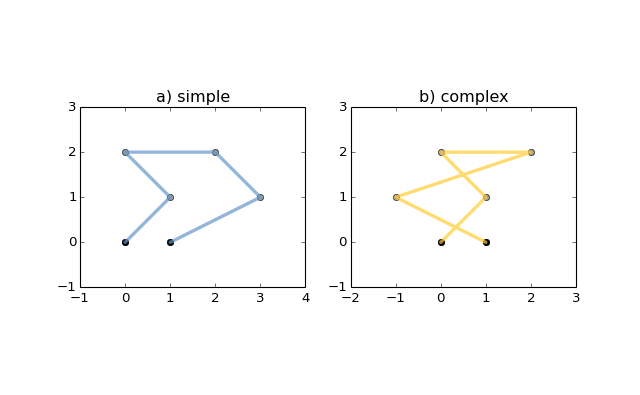Figure 1. A simpleLineStringon the left, a complexLineStringon the right. The (MultiPoint) boundary of each is shown in black, the other points that describe the lines are shown in grey.

ALineStringhas zero area and non-zero length.

>>> from shapely.geometry import LineString
>>> line = LineString([(0, 0), (1, 1)])
>>> line.area0.0>>> line.length1.4142135623730951

Itsx-ybounding box is a (minx, miny, maxx, maxy) tuple.

>>> line.bounds(0.0, 0.0, 1.0, 1.0)

The defining coordinate values are accessed via thecoordsproperty.

>>> len(line.coords)2
>>> list(line.coords)[(0.0, 0.0), (1.0, 1.0)]

Coordinates may also be sliced.New in version 1.2.14.

>>> point.coords[:][(0.0, 0.0), (1.0, 1.0)]
>>> point.coords[1:][(1.0, 1.0)]

The constructor also accepts anotherLineStringinstance, thereby making a copy.

>>> LineString(line)
<shapely.geometry.linestring.LineString object at 0x...>

A sequence ofPointinstances is not a valid constructor parameter. ALineStringis described by points, but is not composed ofPointinstances.

### LinearRings

• class LinearRing(coordinates)

• TheLinearRingconstructor takes an ordered sequence of (x, y[, z])point tuples.

The sequence may be explicitly closed by passing identical values in the first and last indices. Otherwise, the sequence will be implicitly closed by copying the first tuple to the last index. As with aLineString, repeated points in the ordered sequence are allowed, but may incur performance penalties and should be avoided. ALinearRingmay not cross itself, and may not touch itself at a single point.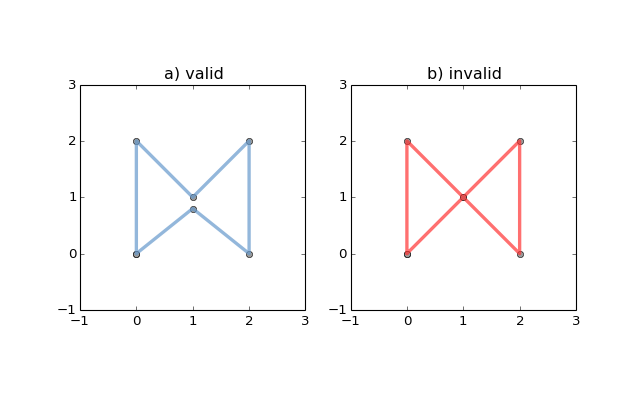Figure 2. A validLinearRingon the left, an invalid self-touchingLinearRingon the right. The points that describe the rings are shown in grey. A ring’s boundary isempty.

Note

Shapely will not prevent the creation of such rings, but exceptions will be raised when they are operated on.

ALinearRinghas zero area and non-zero length.

>>> from shapely.geometry.polygon import LinearRing
>>> ring = LinearRing([(0, 0), (1, 1), (1, 0)])
>>> ring.area0.0
>>> ring.length3.4142135623730949

Itsx-ybounding box is a (minx, miny, maxx, maxy) tuple.

>>> ring.bounds(0.0, 0.0, 1.0, 1.0)

Defining coordinate values are accessed via thecoordsproperty.

>>> len(ring.coords)
4
>>> list(ring.coords)
[(0.0, 0.0), (1.0, 1.0), (1.0, 0.0), (0.0, 0.0)]

TheLinearRingconstructor also accepts anotherLineStringorLinearRinginstance, thereby making a copy.

>>> LinearRring(ring)
<shapely.geometry.polygon.LinearRing object at 0x...>

As withLineString, a sequence ofPointinstances is not a valid constructor parameter.

### Polygons

• class Polygon(exterior[, interiors=None])

• ThePolygonconstructor takes two positional parameters. The first is an ordered sequence of (x, y[, z]) point tuples and is treated exactly as in theLinearRingcase. The second is an optional unordered sequence of ring-like sequences specifying the interior boundaries or “holes” of the feature.

Rings of avalidPolygonmay not cross each other, but may touch at a single point only.  Again, Shapely will not prevent the creation of invalid features, but exceptions will be raised when they are operated on.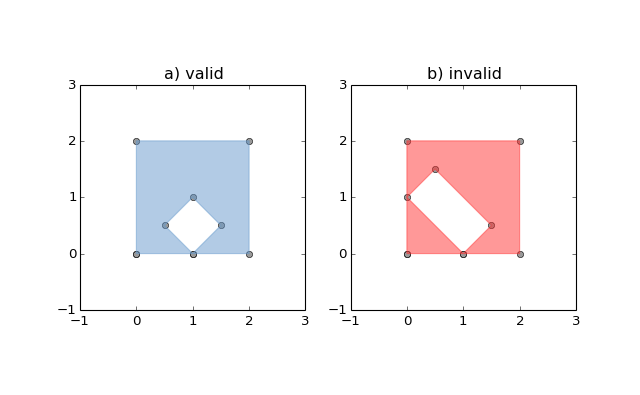Figure 3. On the left, a validPolygonwith one interior ring that touches the exterior ring at one point, and on the right aPolygonthat isinvalidbecause its interior ring touches the exterior ring at more than one point. The points that describe the rings are shown in grey.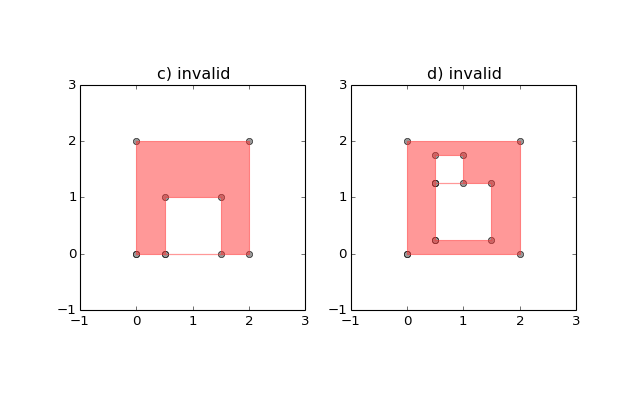Figure 4. On the left, aPolygonthat isinvalidbecause its exterior and interior rings touch along a line, and on the right, aPolygonthat isinvalidbecause its interior rings touch along a line.

APolygonhas non-zero area and non-zero length.

>>> from shapely.geometry import Polygon
>>> polygon = Polygon([(0, 0), (1, 1), (1, 0)])
>>> polygon.area0.5
>>> polygon.length3.4142135623730949

Itsx-ybounding box is a (minx, miny, maxx, maxy) tuple.

>>> polygon.bounds(0.0, 0.0, 1.0, 1.0)

Component rings are accessed viaexteriorandinteriorsproperties.

>>> list(polygon.exterior.coords)
[(0.0, 0.0), (1.0, 1.0), (1.0, 0.0), (0.0, 0.0)]
>>> list(polygon.interiors)
[]

ThePolygonconstructor also accepts instances ofLineStringandLinearRing.

>>> coords = [(0, 0), (1, 1), (1, 0)]
>>> r = LinearRing(coords)>>> s = Polygon(r)
>>> s.area0.5
>>> t = Polygon(s.buffer(1.0).exterior, [r])
>>> t.area6.5507620529190334

Rectangular polygons occur commonly, and can be conveniently constructed using the shapely.geometry.box() function.

• shapely.geometry.box(minx, miny, maxx, maxy, ccw=True)

• Makes a rectangular polygon from the provided bounding box values, with counter-clockwise order by default.

New in version 1.2.9.

For example:

>>> from shapely.geometry import box
>>> b = box(0.0, 0.0, 1.0, 1.0)
>>> b
<shapely.geometry.polygon.Polygon object at 0x...
>>>> list(b.exterior.coords)
[(1.0, 0.0), (1.0, 1.0), (0.0, 1.0), (0.0, 0.0), (1.0, 0.0)]

This is the first appearance of an explicit polygon handedness in Shapely.

To obtain a polygon with a known orientation, useshapely.geometry.polygon.orient():

• shapely.geometry.polygon.orient(polygon, sign=1.0)

• Returns a properly oriented copy of the given polygon. The signed area of the result will have the given sign. A sign of 1.0 means that the coordinates of the product’s exterior ring will be oriented counter-clockwise.

New in version 1.2.10.

### Collections

Heterogeneous collections of geometric objects may result from some Shapely operations. For example, twoLineStringsmay intersect along a line and at a point. To represent these kind of results, Shapely provides frozenset-like, immutable collections of geometric objects.  The collections may be homogeneous (MultiPointetc.) or heterogeneous.

>>> a = LineString([(0, 0), (1, 1), (1,2), (2,2)])
>>> b = LineString([(0, 0), (1, 1), (2,1), (2,2)])
>>> x = a.intersection(b)
>>> x
<shapely.geometry.collection.GeometryCollection object at 0x...

>>>> from pprint import pprint
>>> pprint(list(x))
[<shapely.geometry.point.Point object at 0x...>,
<shapely.geometry.linestring.LineString object at 0x...>]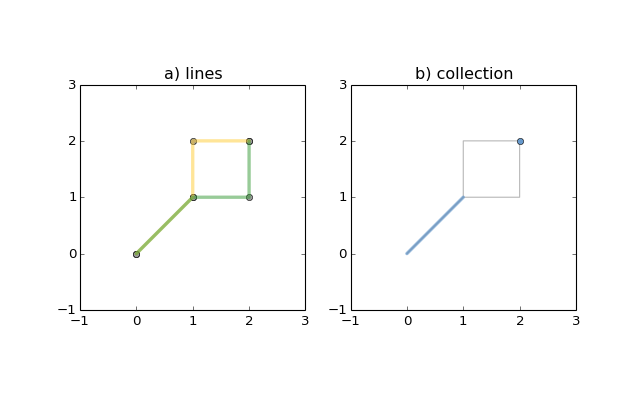Figure 5. a) a green and a yellow line that intersect along a line and at a single point; b) the intersection (in blue) is a collection containing oneLineStringand onePoint.

Members of aGeometryCollectionare accessed via thegeomsproperty or via the iterator protocol using in  or list().

>>> pprint(list(x.geoms))
[<shapely.geometry.point.Point object at 0x...>,
<shapely.geometry.linestring.LineString object at 0x...>]

>>> pprint(list(x))
[<shapely.geometry.point.Point object at 0x...>,
<shapely.geometry.linestring.LineString object at 0x...>]

Homogeneous collections can also be sliced, resulting in a new object of the same type.

>>> from shapely.geometry import MultiPoint
>>> m = MultiPoint([(0, 0), (1, 1), (1,2), (2,2)])

>>> m[:1].wkt
'MULTIPOINT (0.0000000000000000 0.0000000000000000)'

>>> m[3:].wkt
'MULTIPOINT (2.0000000000000000 2.0000000000000000)'

>>> m[4:].wkt'GEOMETRYCOLLECTION EMPTY'

New in version 1.2.14.

Note

When possible, it is better to use one of the homogeneous collection types described below.

### Collections of Points

• class MultiPoint(points)

• TheMultiPointconstructor takes a sequence of (x, y[, z ]) point tuples.

AMultiPointhas zero area and zero length.

>>> from shapely.geometry import MultiPoint
>>> points = MultiPoint([(0.0, 0.0), (1.0, 1.0)])
>>> points.area
0.0
>>> points.length
0.0

Itsx-ybounding box is a (minx, miny, maxx, maxy) tuple.

>>> points.bounds(0.0, 0.0, 1.0, 1.0)

Members of a multi-point collection are accessed via the geoms property or via the iterator protocol using in or list().

>>> import pprint
>>> pprint.pprint(list(points.geoms))
[<shapely.geometry.point.Point object at 0x...>,
<shapely.geometry.point.Point object at 0x...>]

>>> pprint.pprint(list(points))
[<shapely.geometry.point.Point object at 0x...>,
<shapely.geometry.point.Point object at 0x...>]

The constructor also accepts anotherMultiPointinstance or an unordered sequence ofPointinstances, thereby making copies.

>>> MultiPoint([Point(0, 0), Point(1, 1)])
<shapely.geometry.multipoint.MultiPoint object at 0x...>

### Collections of Lines

• class MultiLineString(lines)

• TheMultiLineStringconstructor takes a sequence of line-like sequences or objects.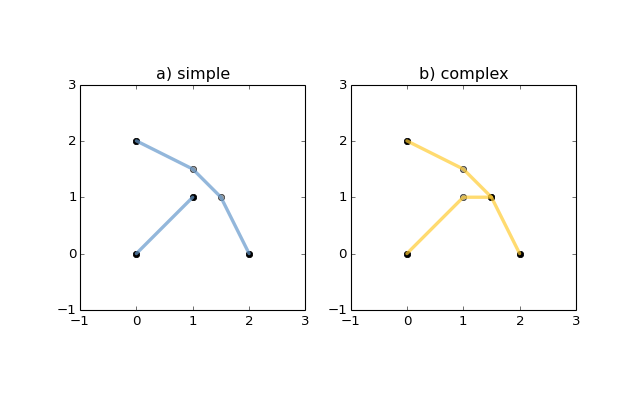Figure 6. On the left, asimple, disconnectedMultiLineString, and on the right, a non-simpleMultiLineString. The points defining the objects are shown in gray, the boundaries of the objects in black.

AMultiLineStringhas zero area and non-zero length.

>>> from shapely.geometry import MultiLineString

>>> coords = [((0, 0), (1, 1)), ((-1, 0), (1, 0))]
>>> lines = MultiLineString(coords)
>>> lines.area0.0
>>> lines.length
3.4142135623730949

Itsx-ybounding box is a (minx, miny, maxx, maxy) tuple.

>>> lines.bounds(-1.0, 0.0, 1.0, 1.0)

Its members are instances ofLineStringand are accessed via the geomsproperty or via the iterator protocol using in or list().

>>> len(lines.geoms)
2
>>> pprint.pprint(list(lines.geoms))
[<shapely.geometry.linestring.LineString object at 0x...>,
<shapely.geometry.linestring.LineString object at 0x...>]

>>> pprint.pprint(list(lines))
[<shapely.geometry.linestring.LineString object at 0x...>,
<shapely.geometry.linestring.LineString object at 0x...>]

The constructor also accepts another instance ofMultiLineStringor an unordered sequence ofLineStringinstances, thereby making copies.

>>> MultiLineString(lines)
<shapely.geometry.multilinestring.MultiLineString object at 0x...

>>>> MultiLineString(lines.geoms)
<shapely.geometry.multilinestring.MultiLineString object at 0x...>

### Collections of Polygons

• class MultiPolygon(polygons)

• TheMultiPolygonconstructor takes a sequence of exterior ring and hole list tuples: [((a1, ..., aM), [(b1, ..., bN), ...]), ...].

More clearly, the constructor also accepts an unordered sequence ofPolygoninstances, thereby making copies.

>>> polygons = MultiPolygon([polygon, s, t])
>>> len(polygons.geoms)
3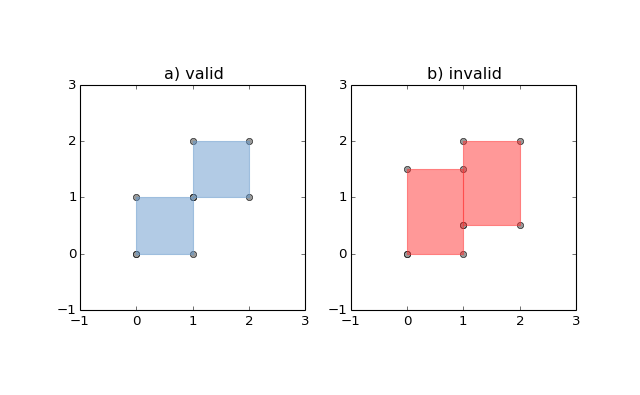Figure 7. On the right, avalidMultiPolygonwith 2 members, and on the right, aMultiPolygonthat is invalid because its members touch at an infinite number of points (along a line).

Itsx-ybounding box is a (minx, miny, maxx, maxy) tuple.

>>> polygons.bounds(-1.0, -1.0, 2.0, 2.0)

Its members are instances ofPolygonand are accessed via the geomsproperty or via the iterator protocol using in or list().

>>> len(polygons.geoms)
3
>>> len(polygons)
3

### Empty features

An “empty” feature is one with a point set that coincides with the empty set; not None, but like set([]). Empty features can be created by calling the various constructors with no arguments. Almost no operations are supported by empty features.

>>> line = LineString()
>>> line.is_emptyTrue
>>> line.length
0.0
>>> line.bounds()
>>> line.coords[]

The coordinates of a empty feature can be set, after which the geometry is no longer empty.

>>> line.coords = [(0, 0), (1, 1)]
>>> line.is_emptyFalse
>>> line.length1.4142135623730951
>>> line.bounds(0.0, 0.0, 1.0, 1.0)

### Linear Referencing Methods

It can be useful to specify position along linear features such asLineStringsandMultiLineStringswith a 1-dimensional referencing system. Shapely supports linear referencing based on length or distance, evaluating the distance along a geometric object to the projection of a given point, or the point at a given distance along the object.

Note

Linear referencing methods require GEOS 3.2.0 or later.

• object.interpolate(distance[, normalized=False])

• Return a point at the specified distance along a linear geometric object.

If thenormalizedarg is True, the distance will be interpreted as a fraction of the geometric object’s length.

>>> ip = LineString([(0, 0), (0, 1), (1, 1)]).interpolate(1.5)
>>> ip<shapely.geometry.point.Point object at 0x740570

>>>> ip.wkt
'POINT (0.5000000000000000 1.0000000000000000)'

>>> LineString([(0, 0), (0, 1), (1, 1)]).interpolate(0.75, normalized=True).wkt
'POINT (0.5000000000000000 1.0000000000000000)'
• object.project(other[, normalized=False])

• Returns the distance along this geometric object to a point nearest theotherobject.

If thenormalizedarg is True, return the distance normalized to the length of the object. The project() method is the inverse ofinterpolate().

>>> LineString([(0, 0), (0, 1), (1, 1)]).project(ip)
1.5
>>> LineString([(0, 0), (0, 1), (1, 1)]).project(ip, normalized=True)
0.75

For example, the linear referencing methods might be used to cut lines at a specified distance.

def cut(line, distance):
# Cuts a line in two at a distance from its starting point
if distance <= 0.0 or distance >= line.length:
return [LineString(line)]
coords = list(line.coords)
for i, p in enumerate(coords):
pd = line.project(Point(p))
if pd == distance:
return [
LineString(coords[:i+1]),
LineString(coords[i:])]
if pd > distance:
cp = line.interpolate(distance)
return [
LineString(coords[:i] + [(cp.x, cp.y)]),
LineString([(cp.x, cp.y)] + coords[i:])]
>>> line = LineString([(0, 0), (1, 0), (2, 0), (3, 0), (4, 0), (5, 0)])

>>> pprint([list(x.coords) for x in cut(line, 1.0)])
[[(0.0, 0.0), (1.0, 0.0)], [(1.0, 0.0), (2.0, 0.0), (3.0, 0.0), (4.0, 0.0), (5.0, 0.0)]]

>>> pprint([list(x.coords) for x in cut(line, 2.5)])
[[(0.0, 0.0), (1.0, 0.0), (2.0, 0.0), (2.5, 0.0)], [(2.5, 0.0), (3.0, 0.0), (4.0, 0.0), (5.0, 0.0)]]

## Predicates and Relationships

Objects of the types explained in Geometric Objects provide standard predicates as attributes (for unary predicates) and methods (for binary predicates). Whether unary or binary, all return True or False.

### Unary Predicates

Standard unary predicates are implemented as read-only property attributes. An example will be shown for each.

• object.has_z

• Returns True if the feature has not onlyxandy, but alsozcoordinates for 3D (or so-called, 2.5D) geometries.

>>> Point(0, 0).has_z
False

>>> Point(0, 0, 0).has_z
True
• object.is_ccw

• Returns True if coordinates are in counter-clockwise order (bounding a region with positive signed area). This method applies toLinearRingobjects only.

New in version 1.2.10.

>>> LinearRing([(1,0), (1,1), (0,0)]).is_ccwTrue

A ring with an undesired orientation can be reversed like this:

>>> ring = LinearRing([(0,0), (1,1), (1,0)])
>>> ring.is_ccwFalse
>>> ring.coords = list(ring.coords)[::-1]
>>> ring.is_ccwTrue
• object.is_empty

• Returns True if the feature’sinteriorandboundary(in point set terms) coincide with the empty set.

>>> Point().is_empty
True

>>> Point(0, 0).is_empty
False

Note

With the help of the operator module’s attrgetter() function, unary predicates such as is_empty can be easily used as predicates for the built in filter() or itertools.ifilter().

>>> from operator import attrgetter
>>> empties = filter(attrgetter('is_empty'), [Point(), Point(0, 0)])
>>> len(empties)1
• object.is_ring

• Returns True if the feature is closed. A closed feature’sboundarycoincides with the empty set.

>>> LineString([(0, 0), (1, 1), (1, -1)]).is_ring
False
>>> LinearRing([(0, 0), (1, 1), (1, -1)]).is_ring
True

This property is applicable toLineStringandLinearRinginstances, but meaningless for others.

• object.is_simple

• Returns True if the feature does not cross itself.

Note

The simplicity test is meaningful only forLineStringsandLinearRings.

>>> LineString([(0, 0), (1, 1), (1, -1), (0, 1)]).is_simple
False

Operations on non-simpleLineStringsare fully supported by Shapely.

• object.is_valid

• Returns True if a feature is “valid” in the sense of .

A validLinearRingmay not cross itself or touch itself at a single point. A validPolygonmay not possess any overlapping exterior or interior rings. A validMultiPolygonmay not collect any overlapping polygons. Operations on invalid features may fail.

>>> MultiPolygon([Point(0, 0).buffer(2.0), Point(1, 1).buffer(2.0)]).is_valid
False

The two points above are close enough that the polygons resulting from the buffer operations (explained in a following section) overlap.

Note

The is_valid predicate can be used to write a validating decorator that could ensure that only valid objects are returned from a constructor function.

from functools import wrapsdef validate(func):
@wraps(func)
def wrapper(*args, **kwargs):
ob = func(*args, **kwargs)
if not ob.is_valid:
raise TopologicalError(
"Given arguments do not determine a valid geometric object")
return ob
return wrapper
>>> @validate
... def ring(coordinates):
...     return LinearRing(coordinates)...

>>> coords = [(0, 0), (1, 1), (1, -1), (0, 1)]
>>> ring(coords)
Traceback (most recent call last):
File "<stdin>", line 1, in <module>
File "<stdin>", line 7, in wrappershapely.geos.TopologicalError:
Given arguments do not determine a valid geometric object

### Binary Predicates

Standard binary predicates are implemented as methods. These predicates evaluate topological, set-theoretic relationships. In a few cases the results may not be what one might expect starting from different assumptions. All take another geometric object as argument and return True or False.

• object.almost_equals(other[, decimal=6])

• Returns True if the object is approximately equal to theotherat all points to specifieddecimalplace precision.

• object.contains(other)

• Returns True if the object’sinteriorcontains theboundaryandinteriorof the other object and their boundaries do not touch at all.

This predicate applies to all types, and is inverse to within(). The expression a.contains(b) == b.within(a) always evaluates to True.

>>> coords = [(0, 0), (1, 1)]
>>> LineString(coords).contains(Point(0.5, 0.5))
True
>>> Point(0.5, 0.5).within(LineString(coords))
True

A line’s endpoints are part of itsboundaryand are therefore not contained.

>>> LineString(coords).contains(Point(1.0, 1.0))
False

Note

Binary predicates can be used directly as predicates for filter() oritertools.ifilter().

>>> line = LineString(coords)
>>> contained = filter(line.contains, [Point(), Point(0.5, 0.5)])
>>> len(contained)
1
>>> [p.wkt for p in contained]['POINT (0.5000000000000000 0.5000000000000000)']
• object.crosses(other)

• Returns True if theinteriorof the object intersects theinteriorof the other but does not contain it, and the dimension of the intersection is less than the dimension of the one or the other.

>>> LineString(coords).crosses(LineString([(0, 1), (1, 0)]))
True

A line does not cross a point that it contains.

>>> LineString(coords).crosses(Point(0.5, 0.5))
False
• object.disjoint(other)

• Returns True if theboundaryandinteriorof the object do not intersect at all with those of the other.

>>> Point(0, 0).disjoint(Point(1, 1))
True

This predicate applies to all types and is the inverse of intersects().

• object.equals(other)

• Returns True if the set-theoreticboundary,interior, andexteriorof the object coincide with those of the other.

The coordinates passed to the object constructors are of these sets, and determine them, but are not the entirety of the sets. This is a potential “gotcha” for new users.  Equivalent lines, for example, can be constructed differently.

>>> a = LineString([(0, 0), (1, 1)])>>> b = LineString([(0, 0), (0.5, 0.5), (1, 1)])>>> c = LineString([(0, 0), (0, 0), (1, 1)])>>> a.equals(b)True>>> b.equals(c)True

This predicate should not be mistaken for Python’s == or isconstructions.

• object.intersects(other)

• Returns True if theboundaryandinteriorof the object intersect in any way with those of the other.

This predicate is equivalent to the OR-ing of contains(), crosses(),equals(), touches(), and within().

• object.touches(other)

• Returns True if the objects have at least one point in common and their interiors do not intersect with any part of the other.

Overlapping features do not thereforetouch, another potential “gotcha”. For example, the following lines touch at (1, 1), but do not overlap.

>>> a = LineString([(0, 0), (1, 1)])
>>> b = LineString([(1, 1), (2, 2)])
>>> a.touches(b)True
• object.within(other)

• Returns True if the object’sboundaryandinteriorintersect only with theinteriorof the other (not itsboundaryorexterior).

This applies to all types and is the inverse of contains().

Used in a sorted()key, within() makes it easy to spatially sort objects. Let’s say we have 4 stereotypic features: a point that is contained by a polygon which is itself contained by another polygon, and a free spirited point contained by none

>>> a = Point(2, 2)
>>> b = Polygon([[1, 1], [1, 3], [3, 3], [3, 1]])
>>> c = Polygon([[0, 0], [0, 4], [4, 4], [4, 0]])
>>> d = Point(-1, -1)

and that copies of these are collected into a list

>>> features = [c, a, d, b, c]

that we’d prefer to have ordered as [d, c, c, b, a] in reverse containment order. As explained in the Python Sorting HowTo, we can define a key function that operates on each list element and returns a value for comparison. Our key function will be a wrapper class that implements __lt__() using Shapely’s binary within() predicate.

from shapely.geometry import asShapeclass Within(object):
def __init__(self, o):
self.o = o
def __lt__(self, other):
return self.o.within(other.o)

As the howto says, theless thancomparison is guaranteed to be used in sorting. That’s what we’ll rely on to spatially sort, and the reason why we usewithin() in reverse instead of contains(). Trying it out on featuresdandc, we see that it works.

>>> d < cTrue
>>> Within(d) < Within(c)
False

It also works on the list of features, producing the order we want.

>>> [d, c, c, b, a] == sorted(features, key=Within, reverse=True)
True

### DE-9IM Relationships

The relate() method tests all the DE-9IM  relationships between objects, of which the named relationship predicates above are a subset.

• object.relate(other)

• Returns a string representation of the DE-9IM matrix of relationships between an object’sinterior,boundary,exteriorand those of another geometric object.

The named relationship predicates (contains(), etc.) are typically implemented as wrappers around relate().

Two different points have mainly F (false) values in their matrix; the intersection of theirexternalsets (the 9th element) is a 2 dimensional object (the rest of the plane). The intersection of theinteriorof one with theexteriorof the other is a 0 dimensional object (3rd and 7th elements of the matrix).

>>> Point(0, 0).relate(Point(1, 1))
'FF0FFF0F2'

The matrix for a line and a point on the line has more “true” (not F) elements.

>>> Point(0, 0).relate(LineString([(0, 0), (1, 1)]))
'F0FFFF102'

Further discussion of the DE-9IM matrix is beyond the scope of this manual. See and http://pypi.python.org/pypi/de9im.

## Spatial Analysis Methods

As well as boolean attributes and methods, Shapely provides analysis methods that return new geometric objects.

### Set-theoretic Methods

Almost every binary predicate method has a counterpart that returns a new geometric object. In addition, the set-theoreticboundaryof an object is available as a read-only attribute.

• object.boundary

• Returns a lower dimensional object representing the object’s set-theoreticboundary.

The boundary of a polygon is a line, the boundary of a line is a collection of points. The boundary of a point is an empty (null) collection.

>> coords = [((0, 0), (1, 1)), ((-1, 0), (1, 0))]
>>> lines = MultiLineString(coords)
>>> lines.boundary<shapely.geometry.multipoint.MultiPoint object at 0x...>
>>> pprint(list(lines.boundary))
[<shapely.geometry.point.Point object at 0x...>,
<shapely.geometry.point.Point object at 0x...>,
<shapely.geometry.point.Point object at 0x...>,
<shapely.geometry.point.Point object at 0x...>]
>>> lines.boundary.boundary<shapely.geometry.collection.GeometryCollection object at 0x...>
>>> lines.boundary.boundary.is_emptyTrue

See the figures in LineStrings and Collections of Lines for the illustration of lines and their boundaries.

• object.centroid

• Returns a representation of the object’s geometric centroid (point).

>>> LineString([(0, 0), (1, 1)]).centroid<shapely.geometry.point.Point object at 0x...>
>>> LineString([(0, 0), (1, 1)]).centroid.wkt
'POINT (0.5000000000000000 0.5000000000000000)'

Note

The centroid of an object might be one of its points, but this is not guaranteed.

• object.difference(other)

• Returns a representation of the points making up this geometric object that do not make up the other object.

>>> a = Point(1, 1).buffer(1.5)
>>> b = Point(2, 1).buffer(1.5)
>>> a.difference(b)
<shapely.geometry.polygon.Polygon object at 0x...>

Note

The buffer() method is used to produce approximately circular polygons in the examples of this section; it will be explained in detail later in this manual.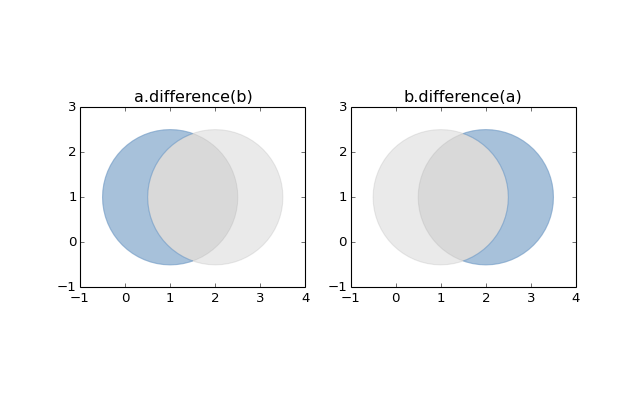Figure 8. Differences between two approximately circular polygons.

Note

Shapely can not represent the difference between an object and a lower dimensional object (such as the difference between a polygon and a line or point) as a single object, and in these cases the difference method returns a copy of the object named self.

• object.intersection(other)

• Returns a representation of the intersection of this object with theothergeometric object.

>>> a = Point(1, 1).buffer(1.5)
>>> b = Point(2, 1).buffer(1.5)
>>> a.intersection(b)
<shapely.geometry.polygon.Polygon object at 0x...>

See the figure under symmetric_difference() below.

• object.symmetric_difference(other)

• Returns a representation of the points in this object not in theothergeometric object, and the points in theothernot in this geometric object.

>>> a = Point(1, 1).buffer(1.5)
>>> b = Point(2, 1).buffer(1.5)
>>> a.symmetric_difference(b)
<shapely.geometry.multipolygon.MultiPolygon object at ...>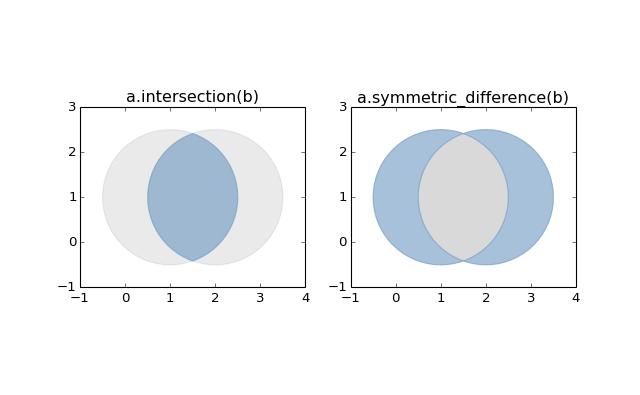• object.union(other)

• Returns a representation of the union of points from this object and theothergeometric object.

The type of object returned depends on the relationship between the operands. The union of polygons (for example) will be a polygon or a multi-polygon depending on whether they intersect or not.

>>> a = Point(1, 1).buffer(1.5)
>>> b = Point(2, 1).buffer(1.5)
>>> a.union(b)
<shapely.geometry.polygon.Polygon object at 0x...>

The semantics of these operations vary with type of geometric object.  For example, compare the boundary of the union of polygons to the union of their boundaries.

>>> a.union(b).boundary
<shapely.geometry.polygon.LinearRing object at 0x...>

>>> a.boundary.union(b.boundary)
<shapely.geometry.multilinestring.MultiLineString object at 0x...>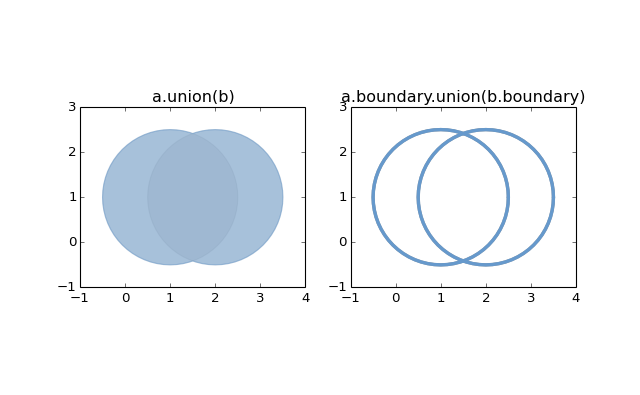Note

union() is an expensive way to find the cumulative union of many objects. See shapely.ops.cascaded_union() for a more effective method.

### Constructive Methods

Shapely geometric object have several methods that yield new objects not derived from set-theoretic analysis.

• object.buffer(distance, resolution=16, cap_style=1, join_style=1, mitre_limit=1.0)

• Returns an approximate representation of all points within a givendistanceof the this geometric object.

The styles of caps are specified by integer values: 1 (round), 2 (flat), 3 (square). These values are also enumerated by the objectshapely.geometry.CAP_STYLE (see below).

The styles of joins between offset segments are specified by integer values: 1 (round), 2 (mitre), and 3 (bevel). These values are also enumerated by the object shapely.geometry.JOIN_STYLE (see below).

• shapely.geometry.CAP_STYLE

• Attribute Value
round 1
flat 2
square 3
• shapely.geometry.JOIN_STYLE

• Attribute Value
round 1
mitre 2
bevel 3
>>> from shapely.geometry import CAP_STYLE, JOIN_STYLE
>>> CAP_STYLE.flat2
>>> JOIN_STYLE.bevel3

A positive distance has an effect of dilation; a negative distance, erosion. The optionalresolutionargument determines the number of segments used to approximate a quarter circle around a point.

>>> line = LineString([(0, 0), (1, 1), (0, 2), (2, 2), (3, 1), (1, 0)])
>>> dilated = line.buffer(0.5)
>>> eroded = dilated.buffer(-0.3)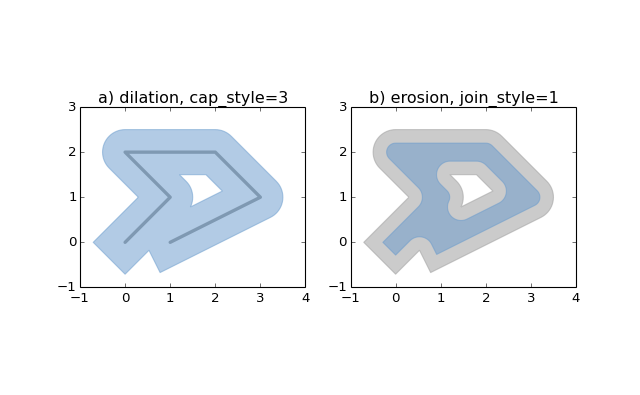Figure 9. Dilation of a line (left) and erosion of a polygon (right). New object is shown in blue.

The default (resolutionof 16) buffer of a point is a polygonal patch with 99.8% of the area of the circular disk it approximates.

>>> p = Point(0, 0).buffer(10.0)
>>> len(p.exterior.coords)66
>>> p.area313.65484905459385

With aresolutionof 1, the buffer is a square patch.

>>> q = Point(0, 0).buffer(10.0, 1)
>>> len(q.exterior.coords)
5
>>> q.area200.0

Passed adistanceof 0, buffer() can be used to “clean” self-touching or self-crossing polygons such as the classic “bowtie”.

>>> coords = [(0, 0), (0, 2), (1, 1), (2, 2), (2, 0), (1, 1), (0, 0)]
>>> bowtie = Polygon(coords)
>>> bowtie.is_validFalse
>>> clean = bowtie.buffer(0)
>>> clean.is_validTrue
>>> clean
<shapely.geometry.multipolygon.MultiPolygon object at ...>
>>> len(clean)
2

>>> list(clean.exterior.coords)
[(0.0, 0.0), (0.0, 2.0), (1.0, 1.0), (0.0, 0.0)]
>>> list(clean.exterior.coords)
[(1.0, 1.0), (2.0, 2.0), (2.0, 0.0), (1.0, 1.0)]

Buffering splits the polygon in two at the point where they touch.

• object.convex_hull

• Returns a representation of the smallest convexPolygoncontaining all the points in the object unless the number of points in the object is less than three. For two points, the convex hull collapses to aLineString; for 1, aPoint.

>>> Point(0, 0).convex_hull
<shapely.geometry.point.Point object at 0x...>
>>> MultiPoint([(0, 0), (1, 1)]).convex_hull
<shapely.geometry.linestring.LineString object at 0x...>

>>> MultiPoint([(0, 0), (1, 1), (1, -1)]).convex_hull
<shapely.geometry.polygon.Polygon object at 0x...>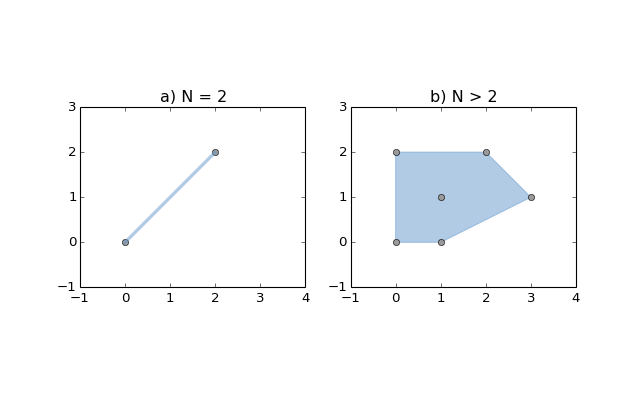Figure 10. Convex hull (blue) of 2 points (left) and of 6 points (right).

• object.envelope

• Returns a representation of the point or smallest rectangular polygon (with sides parallel to the coordinate axes) that contains the object.

>>> Point(0, 0).envelope<shapely.geometry.point.Point object at 0x...>>>> MultiPoint([(0, 0), (1, 1)]).envelope<shapely.geometry.polygon.Polygon object at 0x...>
• object.parallel_offset(distance, side, resolution=16, join_style=1, mitre_limit=1.0)

• Returns a LineString or MultiLineString geometry at a distance from the object on its right or its left side.

Distance must be a positive float value. The side parameter may be ‘left’ or ‘right’. The resolution of the offset around each vertex of the object is parameterized as in the buffer method.

The join style is for outside corners between line segments. Accepted integer values are 1 (round), 2 (mitre), and 3 (bevel). See alsoshapely.geometry.JOIN_STYLE.

Severely mitered corners can be controlled by the mitre_limit parameter (spelled in British English, en-gb). The ratio of the distance from the corner to the end of the mitred offset corner is the miter ratio. Corners with a ratio which exceed the limit will be beveled.

Note

This method is only available forLinearRingandLineStringobjects.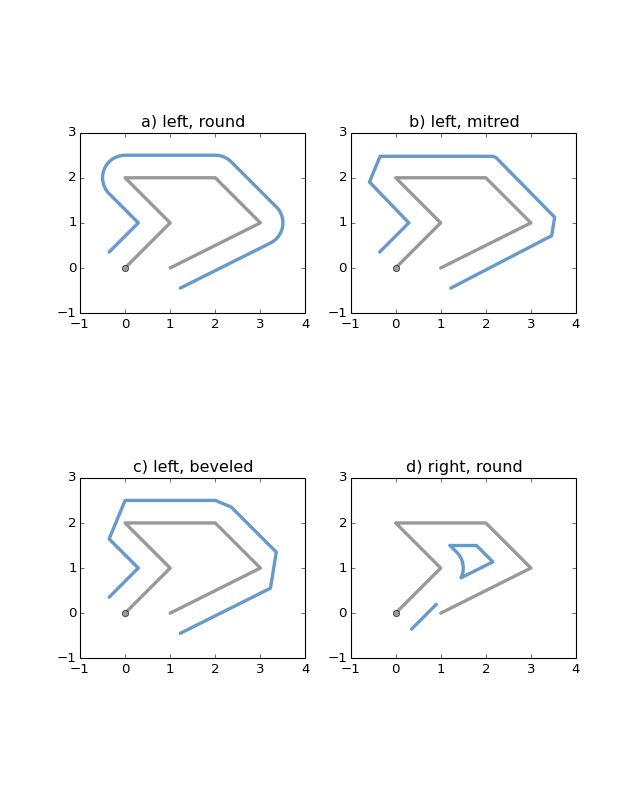Figure 11. Three styles of parallel offset lines on the left side of a simple line string (its starting point shown as a circle) and one offset on the right side, a multipart.

The effect of themitre_limitparameter is shown below.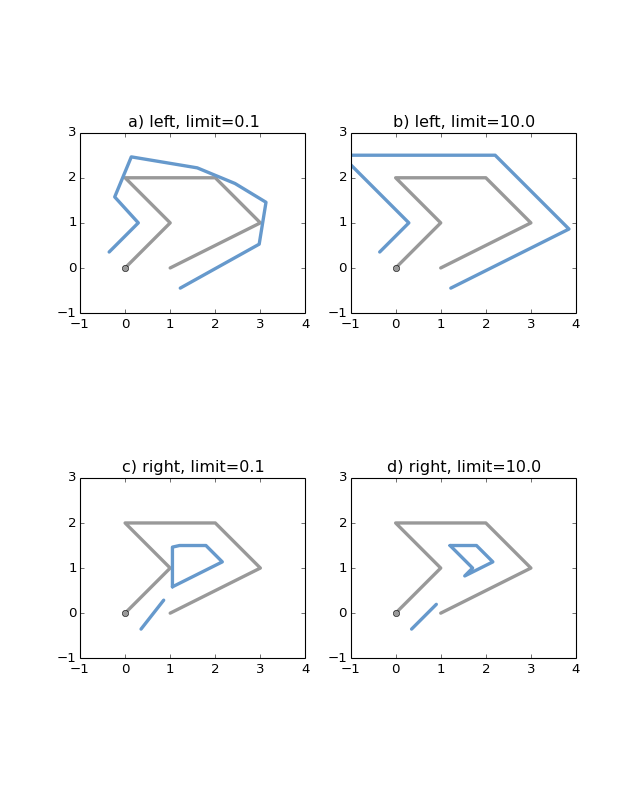Figure 12. Large and small mitre_limit values for left and right offsets.

• object.simplify(tolerance, preserve_topology=True)

• Returns a simplified representation of the geometric object.

All points in the simplified object will be within thetolerancedistance of the original geometry. By default a slower algorithm is used that preserves topology. If preserve topology is set to False the much quicker Douglas-Peucker algorithm  is used.

>>> p = Point(0.0, 0.0)
>>> x = p.buffer(1.0)
>>> x.area
3.1365484905459389
>>> len(x.exterior.coords)
66
>>> s = x.simplify(0.05, preserve_topology=False)
>>> s.area
3.0614674589207187
>>> len(s.exterior.coords)
17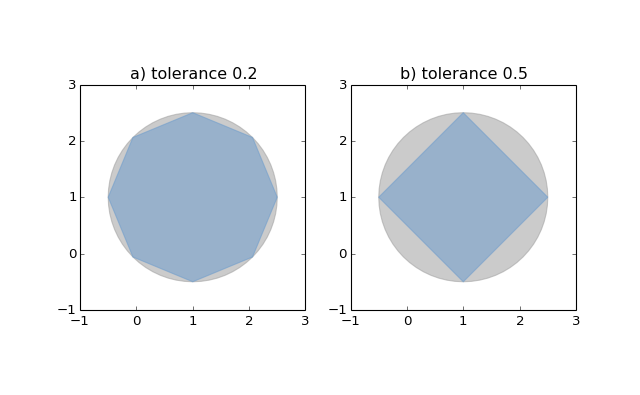Figure 13. Simplification of a nearly circular polygon using a tolerance of 0.2 (left) and 0.5 (right).

Note

Invalidgeometric objects may result from simplification that does not preserve topology.

## Affine Transformations

A collection of affine transform functions are in the shapely.affinitymodule, which return transformed geometries by either directly supplying coefficients to an affine transformation matrix, or by using a specific, named transform (rotate,scale, etc.). The functions can be used with all geometry types (exceptGeometryCollection), and 3D types are either preserved or supported by 3D affine transformations.

New in version 1.2.17.

• shapely.affinity.affine_transform(geom, matrix)

• Returns a transformed geometry using an affine transformation matrix.

The coefficient matrix is provided as a list or tuple with 6 or 12 items for 2D or 3D transformations, respectively.

For 2D affine transformations, the 6 parameter matrix is:

[a, b, d, e, xoff, yoff]

which represents the augmented matrix:

\begin{bmatrix} x' & y' & 1 \end{bmatrix} = \begin{bmatrix} x  & y  & 1 \end{bmatrix} \begin{bmatrix}  a & b & x_\mathrm{off} \\  d & e & y_\mathrm{off} \\  0 & 0 & 1 \end{bmatrix}

or the equations for the transformed coordinates:

x' &= a x + b y + x_\mathrm{off} \\ y' &= d x + e y + y_\mathrm{off}.

For 3D affine transformations, the 12 parameter matrix is:

[a, b, c, d, e, f, g, h, i, xoff, yoff, zoff]

which represents the augmented matrix:

\begin{bmatrix} x' & y' & z' & 1 \end{bmatrix} = \begin{bmatrix} x  & y  & z  & 1 \end{bmatrix} \begin{bmatrix}  a & b & c & x_\mathrm{off} \\  d & e & f & y_\mathrm{off} \\  g & h & i & z_\mathrm{off} \\  0 & 0 & 0 & 1 \end{bmatrix}

or the equations for the transformed coordinates:

x' &= a x + b y + c z + x_\mathrm{off} \\ y' &= d x + e y + f z + y_\mathrm{off} \\ z' &= g x + h y + i z + z_\mathrm{off}.

• Returns a rotated geometry on a 2D plane.

The angle of rotation can be specified in either degrees (default) or radians by setting use_radians=True. Positive angles are counter-clockwise and negative are clockwise rotations.

The point of origin can be a keyword 'center' for the bounding box center (default), 'centroid' for the geometry’s centroid, aPointobject or a coordinate tuple (x0, y0).

The affine transformation matrix for 2D rotation with angle \theta is:

\begin{bmatrix}  \cos{\theta} & -\sin{\theta} & x_\mathrm{off} \\  \sin{\theta} &  \cos{\theta} & y_\mathrm{off} \\        0      &        0      & 1 \end{bmatrix}

where the offsets are calculated from the origin (x_0, y_0):

x_\mathrm{off} &= x_0 - x_0 \cos{\theta} + y_0 \sin{\theta} \\ y_\mathrm{off} &= y_0 - x_0 \sin{\theta} - y_0 \cos{\theta}

>>> from shapely import affinity
>>> line = LineString([(1, 3), (1, 1), (4, 1)])
>>> rotated_a = affinity.rotate(line, 90)
>>> rotated_b = affinity.rotate(line, 90, origin='centroid')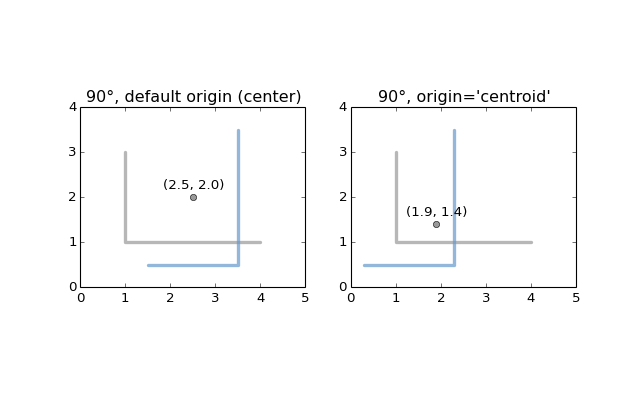Figure 14. Rotation of aLineString(gray) by an angle of 90° counter-clockwise (blue) using different origins.

• shapely.affinity.scale(geom, xfact=1.0, yfact=1.0, zfact=1.0, origin='center')

Returns a scaled geometry, scaled by factors along each dimension.

The point of origin can be a keyword 'center' for the 2D bounding box center (default), 'centroid' for the geometry’s 2D centroid, aPointobject or a coordinate tuple (x0, y0, z0).

Negative scale factors will mirror or reflect coordinates.

The general 3D affine transformation matrix for scaling is:

\begin{bmatrix}  x_\mathrm{fact} & 0               & 0               & x_\mathrm{off} \\  0               & y_\mathrm{fact} & 0               & y_\mathrm{off} \\  0               & 0               & z_\mathrm{fact} & z_\mathrm{off} \\  0               & 0               & 0               & 1 \end{bmatrix}

where the offsets are calculated from the origin (x_0, y_0, z_0):

x_\mathrm{off} &= x_0 - x_0 x_\mathrm{fact} \\ y_\mathrm{off} &= y_0 - y_0 y_\mathrm{fact} \\ z_\mathrm{off} &= z_0 - z_0 z_\mathrm{fact}

>>> triangle = Polygon([(1, 1), (2, 3), (3, 1)])
>>> triangle_a = affinity.scale(triangle, xfact=1.5, yfact=-1)
>>> triangle_a.exterior.coords[:]
[(0.5, 3.0), (2.0, 1.0), (3.5, 3.0), (0.5, 3.0)]

>>> triangle_b = affinity.scale(triangle, xfact=2, origin=(1,1))
>>> triangle_b.exterior.coords[:]
[(1.0, 1.0), (3.0, 3.0), (5.0, 1.0), (1.0, 1.0)]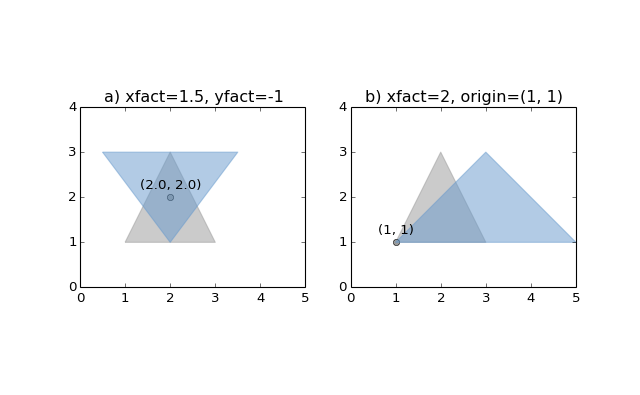Figure 15. Scaling of a gray triangle to blue result: a) by a factor of 1.5 along x-direction, with reflection across y-axis; b) by a factor of 2 along x-direction with custom origin at (1, 1).

• shapely.affinity.skew(geom, xs=0.0, ys=0.0, origin='center', use_radians=False)

• Returns a skewed geometry, sheared by angles along x and y dimensions.

The shear angle can be specified in either degrees (default) or radians by setting use_radians=True.

The point of origin can be a keyword 'center' for the bounding box center (default), 'centroid' for the geometry’s centroid, aPointobject or a coordinate tuple (x0, y0).

The general 2D affine transformation matrix for skewing is:

\begin{bmatrix}  1 & \tan{x_s} & x_\mathrm{off} \\  \tan{y_s} & 1 & y_\mathrm{off} \\  0 & 0 & 1 \end{bmatrix}

where the offsets are calculated from the origin (x_0, y_0):

x_\mathrm{off} &= -y_0 \tan{x_s} \\ y_\mathrm{off} &= -x_0 \tan{y_s}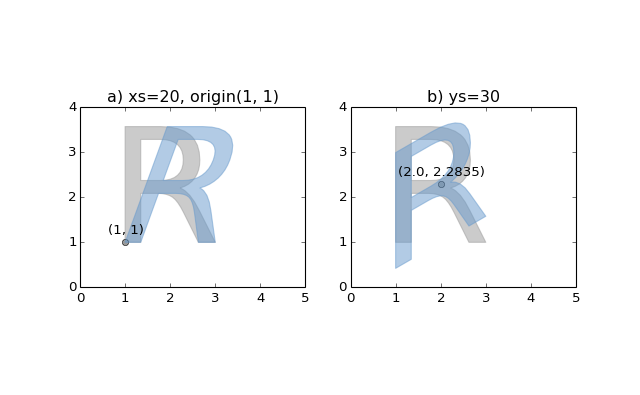Figure 16. Skewing of a gray “R” to blue result: a) by a shear angle of 20° along the x-direction and an origin at (1, 1); b) by a shear angle of 30° along the y-direction, using default origin.

• shapely.affinity.translate(geom, xoff=0.0, yoff=0.0, zoff=0.0)

• Returns a translated geometry shifted by offsets along each dimension.

The general 3D affine transformation matrix for translation is:

\begin{bmatrix}  1 & 0 & 0 & x_\mathrm{off} \\  0 & 1 & 0 & y_\mathrm{off} \\  0 & 0 & 1 & z_\mathrm{off} \\  0 & 0 & 0 & 1 \end{bmatrix}

## Other Transformations

Shapely supports map projections and other arbitrary transformations of geometric objects.

• shapely.ops.transform(func, geom)

• Appliesfuncto all coordinates ofgeomand returns a new geometry of the same type from the transformed coordinates.

funcmaps x, y, and optionally z to output xp, yp, zp. The input parameters may iterable types like lists or arrays or single values. The output shall be of the same type: scalars in, scalars out; lists in, lists out.

New in version 1.2.18.

For example, here is an identity function applicable to both types of input (scalar or array).

def id_func(x, y, z=None):
return tuple(filter(None, [x, y, z]))

g2 = transform(id_func, g1)

A partially applied transform function from pyproj satisfies the requirements forfunc.

from functools import partial
import pyprojproject = partial(
pyproj.transform,
pyproj.Proj(init='espg:4326'),
pyproj.Proj(init='epsg:26913'))

g2 = transform(project, g1)

Lambda expressions such as the one in

g2 = transform(lambda x, y, z=None: (x+1.0, y+1.0), g1)

also satisfy the requirements forfunc.

## Other Operations

### Merging Linear Features

Sequences of touching lines can be merged intoMultiLineStringsorPolygonsusing functions in the shapely.ops module.

• shapely.ops.polygonize(lines)

• Returns an iterator over polygons constructed from the inputlines.

As with the MultiLineString constructor, the input elements may be any line-like object.

>>> from shapely.ops import polygonize
>>> lines = [...     ((0, 0), (1, 1)),...     ((0, 0), (0, 1)),...     ((0, 1), (1, 1)),...     ((1, 1), (1, 0)),...     ((1, 0), (0, 0))...     ]>>> pprint(list(polygonize(lines)))[<shapely.geometry.polygon.Polygon object at 0x...>, <shapely.geometry.polygon.Polygon object at 0x...>]
• shapely.ops.polygonize_full(lines)

Creates polygons from a source of lines, returning the polygons and leftover geometries.

The source may be a MultiLineString, a sequence of LineString objects, or a sequence of objects than can be adapted to LineStrings.

Returns a tuple of objects: (polygons, dangles, cut edges, invalid ring lines). Each are a geometry collection.

Dangles are edges which have one or both ends which are not incident on another edge endpoint. Cut edges are connected at both ends but do not form part of polygon. Invalid ring lines form rings which are invalid (bowties, etc).

New in version 1.2.18.

>>> lines = [
...     ((0, 0), (1, 1)),
...     ((0, 0), (0, 1)),
...     ((0, 1), (1, 1)),
...     ((1, 1), (1, 0)),
...     ((1, 0), (0, 0)),
...     ((5, 5), (6, 6)),
...     ((1, 1), (100, 100)),
...     ]
>>> result, dangles, cuts, invalids = polygonize_full(lines)
>>> len(result)
2
>>> list(result.geoms)
[<shapely.geometry.polygon.Polygon object at ...>,
<shapely.geometry.polygon.Polygon object at ...>]
>>> list(cuts.geoms)
[<shapely.geometry.linestring.LineString object at ...>,
<shapely.geometry.linestring.LineString object at ...>]
• shapely.ops.linemerge(lines)

• Returns aLineStringorMultiLineStringrepresenting the merger of all contiguous elements oflines.

As with shapely.ops.polygonize(), the input elements may be any line-like object.

>>> from shapely.ops import linemerge>>> linemerge(lines)<shapely.geometry.multilinestring.MultiLineString object at 0x...>>>> pprint(list(linemerge(lines)))[<shapely.geometry.linestring.LineString object at 0x...>, <shapely.geometry.linestring.LineString object at 0x...>, <shapely.geometry.linestring.LineString object at 0x...>]

The cascaded_union() function inshapely.opsis more efficient than accumulating with union().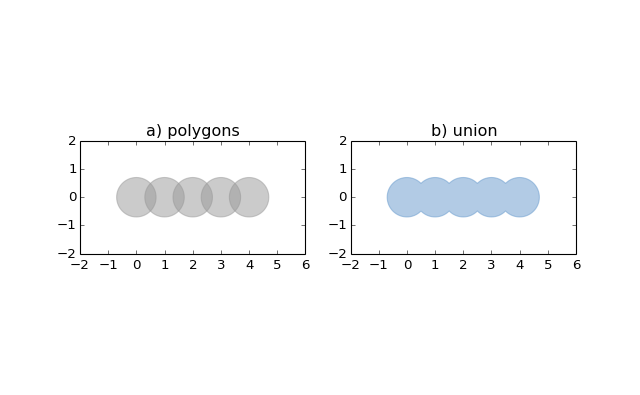Returns a representation of the union of the given geometric objects.

>>> from shapely.ops import cascaded_union
>>> polygons = [Point(i, 0).buffer(0.7) for i in range(5)]
<shapely.geometry.polygon.Polygon object at 0x...>

The function is particularly useful in dissolvingMultiPolygons.

>>> m = MultiPolygon(polygons)
>>> m.area7.6845438018375516
>>> cascaded_union(m).area6.6103013551167971

Note

In 1.2.16 shapely.ops.cascaded_union() is superceded byshapely.ops.unary_union() if GEOS 3.2+ is used. The unary union function can operate on different geometry types, not only polygons as is the case for the older cascaded unions.

• shapely.ops.unary_union(geoms)

• Returns a representation of the union of the given geometric objects.

### Prepared Geometry Operations

Shapely geometries can be processed into a state that supports more efficient batches of operations.

• prepared.prep(ob)

• Creates and returns a prepared geometric object.

To test one polygon containment against a large batch of points, one should first use the prepared.prep() function.

>>> from shapely.geometry import Point
>>> from shapely.prepared import prep
>>> points = [...] # large list of points
>>> polygon = Point(0.0, 0.0).buffer(1.0)
>>> prepared_polygon = prep(polygon)
>>> prepared_polygon
<shapely.prepared.PreparedGeometry object at 0x...>
>>> hits = filter(prepared_polygon.contains, points)

Prepared geometries instances have the following methods: contains,contains_properly, covers, and intersects. All have exactly the same arguments and usage as their counterparts in non-prepared geometric objects.

### Diagnostics

• validation.explain_validity(ob):

• Returns a string explaining the validity or invalidity of the object.

New in version 1.2.1.

The messages may or may not have a representation of a problem point that can be parsed out.

>>> coords = [(0, 0), (0, 2), (1, 1), (2, 2), (2, 0), (1, 1), (0, 0)]
>>> p = Polygon(coords)
>>> from shapely.validation import explain_validity
>>> explain_validity(p)'Ring Self-intersection[1 1]'

The Shapely version, GEOS library version, and GEOS C API version are accessible via shapely.__version__,shapely.geos.geos_version_string, andshapely.geos.geos_capi_version.

>>> import shapely>>> shapely.__version__'1.3.0'
>>> import shapely.geos>>> shapely.geos.geos_version(3, 3, 0)
>>> shapely.geos.geos_version_string'3.3.0-CAPI-1.7.0'

## Interoperation

Shapely provides 4 avenues for interoperation with other software.

### Well-Known Formats

AWell Known Text(WKT) orWell Known Binary(WKB) representation  of any geometric object can be had via its wkt or wkb attribute. These representations allow interchange with many GIS programs. PostGIS, for example, trades in hex-encoded WKB.

>>> Point(0, 0).wkt'POINT (0.0000000000000000 0.0000000000000000)'
>>> Point(0, 0).wkb.encode('hex')'010100000000000000000000000000000000000000'

Theshapely.wktandshapely.wkbmodules providedumps()andloads()functions that work almost exactly as theirpickleandsimplejsonmodule counterparts. To serialize a geometric object to a binary or text string, usedumps(). To deserialize a string and get a new geometric object of the appropriate type, use loads().

• shapely.wkb.dumps(ob)

• Returns a WKB representation ofob.

• Returns a geometric object from a WKB representationwkb.

>> from shapely.wkb import dumps, loads
>>> wkb = dumps(Point(0, 0))
>>> print wkb.encode('hex')
010100000000000000000000000000000000000000
'POINT (0.0000000000000000 0.0000000000000000)'

All of Shapely’s geometry types are supported by these functions.

• shapely.wkt.dumps(ob)

• Returns a WKT representation ofob.

• Returns a geometric object from a WKT representationwkt.

>> wkt = dumps(Point(0, 0))
>>> print wktPOINT (0.0000000000000000 0.0000000000000000)
'POINT (0.0000000000000000 0.0000000000000000)'

### Numpy and Python Arrays

All geometric objects with coordinate sequences (Point,LinearRing,LineString) provide the Numpy array interface and can thereby be converted or adapted to Numpy arrays.

>>> from numpy import array

>>> array(Point(0, 0))
array([ 0.,  0.])

>>> array(LineString([(0, 0), (1, 1)]))
array([[ 0.,  0.],       [ 1.,  1.]])

The numpy.asarray() function does not copy coordinate values – at the price of slower Numpy access to the coordinates of Shapely objects.

Note

The Numpy array interface is provided without a dependency on Numpy itself.

The coordinates of the same types of geometric objects can be had as standard Python arrays ofxandyvalues via the xy attribute.

>>> Point(0, 0).xy(array('d', [0.0]), array('d', [0.0]))
>>> LineString([(0, 0), (1, 1)]).xy(array('d', [0.0, 1.0]), array('d', [0.0, 1.0]))

The shapely.geometry.asShape() family of functions can be used to wrap Numpy coordinate arrays so that they can then be analyzed using Shapely while maintaining their original storage. A 1 x 2 array can be adapted to a point

>>> from shapely.geometry import asPoint
>>> pa = asPoint(array([0.0, 0.0]))
>>> pa.wkt
'POINT (0.0000000000000000 0.0000000000000000)'

and a N x 2 array can be adapted to a line string

>>> from shapely.geometry import asLineString
>>> la = asLineString(array([[1.0, 2.0], [3.0, 4.0]]))
>>> la.wkt
'LINESTRING (1.0000000000000000 2.0000000000000000, 3.0000000000000000 4.0000000000000000)'

There is no Numpy array representation of a polygon.

### Python Geo Interface

Any object that provides the GeoJSON-like Python geo interface can be adapted and used as a Shapely geometry using theshapely.geometry.asShape() or shapely.geometry.shape() functions.

• shapely.geometry.asShape(context)

• Adapts the context to a geometry interface. The coordinates remain stored in the context.

• shapely.geometry.shape(context)

• Returns a new, independent geometry with coordinatescopiedfrom the context.

For example, a dictionary:

>>> from shapely.geometry import asShape
>>> d = {"type": "Point", "coordinates": (0.0, 0.0)}
>>> shape = asShape(d)
>>> shape.geom_type'Point'
>>> list(shape.coords)[(0.0, 0.0)]

Or a simple placemark-type object:

>>> class GeoThing(object):
...     def __init__(self, d):
...         self.__geo_interface__ = d
>>> thing = GeoThing({"type": "Point", "coordinates": (0.0, 0.0)})
>>> shape = asShape(thing)
>>> shape.geom_type'Point'
>>> list(shape.coords)
[(0.0, 0.0)]

The GeoJSON-like mapping of a geometric object can be obtained usingshapely.geometry.mapping().

• shapely.geometry.mapping(ob)

• Returns a new, independent geometry with coordinatescopiedfrom the context.

New in version 1.2.3.

For example, using the sameGeoThingclass:

>>> from shapely.geometry import mapping
>>> thing = GeoThing({"type": "Point", "coordinates": (0.0, 0.0)})
>>> m = mapping(thing)
>>> m['type']'Point'
>>> m['coordinates']
(0.0, 0.0)}

## Performance

Shapely uses the GEOS library for all operations. GEOS is written in C++ and used in many applications and you can expect that all operations are highly optimized. The creation of new geometries with many coordinates, however, involves some overhead that might slow down your code.

New in version 1.2.10.

The shapely.speedups module contains performance enhancements written in C. They are automaticaly installed when Python has access to a compiler and GEOS development headers during installation.

You can check if the speedups are installed with the availableattribute. The constructor speedups are disabled by default. To enable the speedups call enable(). You can revert to the default implementation withdisable().

>>> from shapely import speedups>>> speedups.availableTrue>>> speedups.enable()

## Conclusion

We hope that you will enjoy and profit from using Shapely. Questions and comments are welcome on the GIS-Python email list. This manual will be updated and improved regularly. Its source is available athttp://github.com/Toblerity/Shapely/tree/master/docs/.

## References

  (1, 2, 3, 4, 5) John R. Herring, Ed., “OpenGIS Implementation Specification for Geographic information - Simple feature access - Part 1: Common architecture,” Oct. 2006.
  (1, 2) M.J. Egenhofer and John R. Herring, Categorizing Binary Topological Relations Between Regions, Lines, and Points in Geographic Databases,  Orono, ME: University of Maine, 1991.
  E. Clementini, P. Di Felice, and P. van Oosterom, “A Small Set of Formal Topological Relationships Suitable for End-User Interaction,” Third International Symposium on Large Spatial Databases (SSD). Lecture Notes in Computer Science no. 692, David Abel and Beng Chin Ooi, Eds.,  Singapore: Springer Verlag, 1993, pp. 277-295.
  (1, 2, 3) C. Strobl, “Dimensionally Extended Nine-Intersection Model (DE-9IM),” Encyclopedia of GIS, S. Shekhar and H. Xiong, Eds., Springer, 2008, pp. 240-245. [PDF]
  Martin Davis, “JTS Technical Specifications,” Mar. 2003. [PDF]
  David H. Douglas and Thomas K. Peucker, “Algorithms for the Reduction of the Number of Points Required to Represent a Digitized Line or its Caricature,” Cartographica: The International Journal for Geographic Information and Geovisualization,  vol. 10, Dec. 1973, pp. 112-122.### 作者的其它热门文章

1
0 收藏

0 评论
0 收藏
1